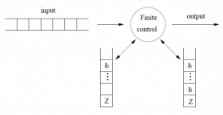Courses

# Test: Multistack Machines, Counter Machines

## 10 Questions MCQ Test Theory of Computation | Test: Multistack Machines, Counter Machines

Description
This mock test of Test: Multistack Machines, Counter Machines for Computer Science Engineering (CSE) helps you for every Computer Science Engineering (CSE) entrance exam. This contains 10 Multiple Choice Questions for Computer Science Engineering (CSE) Test: Multistack Machines, Counter Machines (mcq) to study with solutions a complete question bank. The solved questions answers in this Test: Multistack Machines, Counter Machines quiz give you a good mix of easy questions and tough questions. Computer Science Engineering (CSE) students definitely take this Test: Multistack Machines, Counter Machines exercise for a better result in the exam. You can find other Test: Multistack Machines, Counter Machines extra questions, long questions & short questions for Computer Science Engineering (CSE) on EduRev as well by searching above.
QUESTION: 1

### Can a single tape turing machine be simulated using deterministic 2-stack turing machine?

Solution:

The symbols to left of the head of turing macine being simulated can be stored on the stack while the symbols on the right of the head can be placed on another stack. On each stack, symbols closer to the TM’s head are placed closer to the top of the stack than symbols farther from the TM’s head.

QUESTION: 2

### A ___________ is a multi tape turing machine whose input tape is read only.

Solution:

Counter machines are offline(a multitape turing machine whose input is read only) whose storage tapes are semi-infinite and whose tape symbols contains only two symbols Z and a blank symbol B.

QUESTION: 3

### nstantaneous description of a counter machine can be described using:

Solution:

nstantaneous description of a counter machine can be described by the state, the input tape contents, the position of input head, and the distance of storage heads from the symbol Z. The counter machine can really store a count on each tape and tell if the count is zero.

QUESTION: 4

Which of the following parameters cannot be used to restrict a turing machine?

Solution:

Another procedure to restrict a turing machine is to limit the size of tape alphabet or reduce the number of states. If the tape alphabets, number of tapes or number of states are limited, then there is only a finite number of different turing machine, so the restricted model is more powerful than the original one.

QUESTION: 5

Linear Bounded Automaton is a:

Solution:

Linear Bounded Automaton is a type of Turing Machine where tape is not allowed to move off the portion of the tape containing the input. It is a Turing machine with limited amount of memory.

QUESTION: 6

Statement: Using a two track tape, we can use a semi infinite tape to simulate an infinte tape.

Solution:

A TM with a semi-infinite tape means that there are no cells to the left of the initial head position. A TM with a semi infinite tape simulates a TM with an infinite tape by using a two-track tape.

QUESTION: 7

Which of the following is true with reference to semi-infinite tape using a two track tape?

Solution:

The upper track represents the cells of the original TM that are at the right of the initial head position. The lower track represents the cells to the left of the initial head position, but in reverse order.

QUESTION: 8

Which among the following options are correct?Statement 1: TMs can accept languages that are not accepted by any PDA with one stack.Statement 2: But PDA with two stacks can accept any language that a TM can accept.

Solution:

Both the statements are true. Both the statements are properties of Multistack machines.

QUESTION: 9

A two-way infinite tape turing machine is ________ superior than the basic model of the turing machine in terms of power.

Solution:

A two way infinite tape turing machine is a turing machine with its input tape infinte in both directions, the other component being the same as the basic model.

QUESTION: 10

For a basic turing machine, there exists an equivalent :

Solution:

For a basic TM, there exists a 2-counter, 3-counter and 4-counter machines
We can prove them using Deterministic two stack turing machine.
Counter machine: